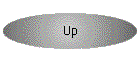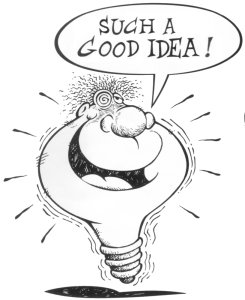Logic Puzzles Geography Quiz Christmas Puzzle Picture Puzzle Monster Quiz

The SSQQ Creativity Test!

 Example: 12 = I in a FAnswer: 12 inches in a foot Example: 24 = H in a D Answer: 24 Hours in a day Example: 365 = D in a Y Answer: 365 days in a year Example: 50 = S in A Answer: 50 States in America

Many of these puzzles are pretty easy.  I knocked off about 20 in the first ten minutes.  Once you get the hang of it, you will probably do even better.  There are currently 102 clues.

If you send me 51 correct answers, I will send you the complete list of all the answers.

 Send your answers by email to dance@ssqq.com    Note: Please put "Creativity Test" in the Subject Line to get your email past the spam controls.  Thanks!Brief Background: In 2001 Ralph Volz sent me a quiz with 57 cluesI decided to add 43 of my own to make a nice round number.  I have to admit as I put together the quiz I had the haunting feeling there were many number clues I was forgetting.

As you work the quiz, I welcome all participants to submit number clues of their own to improve the test!!

01]  1 = F over the C N
02]  2 = Y before the M
03]  3 = C in a F
04]  4 = W and a F
05]  5 = R in the O S
06]  6 = W of H the E
07]  7 = B for S B
08]  8 = S on a S S
09]  9 = I in a B G
10]  10 = A in the B of R
11]  11 = P on a F T
12]  12 = L of H
13]  13 = C in a S
14]Ā 7 = D of the W
15]Ā 15 = is the D C D on the I of M
16]Ā 16 = O in a P
17]Ā 17 = a S by J I
18]Ā 18 = H on a G C
19]Ā 19 = N B by the R S
20]Ā 20 = Y that R V W S
21]Ā 21 = G S
22]Ā 22 = a C N in a J H N
23]  4 = Q in a G
24]Ā 24 = H in a D
25]Ā 200 = D for P G in M
26]Ā 26 = L of the A
27]Ā 27 = B in the N T
28]Ā 15 = M on a D M C
29]Ā 29 = D in F in a L Y
30]Ā 30 = D H S A J and N
31]  31 = I C F at B ¢ R
32]Ā 32 = C in a G of S
33]Ā 33 = R P M on a R A
34]Ā 34 = a S in a F C M
35]  3 = M in the N by A D
36]Ā 36 = I in a Y
37]Ā 18 = W on a S T
38]Ā 38 = S is a R and a R and R B
39]Ā 39 = S (a M by A H)
40]Ā 40 = T (plus A B)
41]Ā 1 = H on a U
42]Ā 9 = J on the SC
43]Ā 43 = P of the U S
44]Ā 21 = D on a D
45]  45 = C R and M L
46]Ā 40 = D and N of the G F
47]Ā 32 = D F at which W F
48]Ā 48 = C in a G of P
49]Ā 212 = D F at which W B
50]Ā 50 = W to L Y L
51]  3 = P for a F G in F

52]Ā 7 = W of the A W
53]Ā 7 = H of R
54]Ā 54 = C in a D (with J)
55]Ā 5 = F on the H
56]Ā 56 = S of the D of I
57]Ā 57 = H V
58]Ā 1 = D at a T
59]  435 = M of the H of R
60]Ā 60 = S in a M
61]Ā 3 = B M (S H T R)
62]  12 = S of the Z
63]Ā 5 = D in a Z C
64]Ā 64 = S on a C B
65]Ā 2 = T D (and a P in a P T)
66]Ā 66 = is the N of a R in a N K C S
67]Ā 12 = K of the R T
68]Ā 4 = H of the A
69]Ā 20,000 = L under the S
70]Ā 70 = is the H R R set by M M
71]  12 = D of J
72]  72 = H in a G T
73]  50 = C in a H D
74]  192 = C in the U N
75]  1001 = A N
76]  76 = T in the B P
77]  77 = S S
78]  13 = original E C
79]  9 = M of A G
80]  80 = D A T W
81]  13 = S on the A F
82]  7 = C on P E
83]  1 = W on a U
84]  32 = T in the A H M
85]  1000 = W that a P is W
86]  451 = D F in a R B B
87]  48 = S on the A C
88]Ā 88 = K on a P
89]  1 = D in the L of I D
90]  90 = D in a R A
91]  9 = P in the SS
92]  56 = G in a R that J D got a H
93]  500 = S of P in a R
94]  1 = G L for M
95]  7 = D and S W
96]  7 = D S
97]  12 = T of I in the B
98]  27 = A to the U S C
99]  99 = B of B on the W
100] 100 = Y W
101] 101 = D
102] 16 = M on a D M C

Can you think of some other clues?  In particular there are numbers like 41, 49, 98 and many more which would be more fun if the number on the quiz matched the number in the quiz.